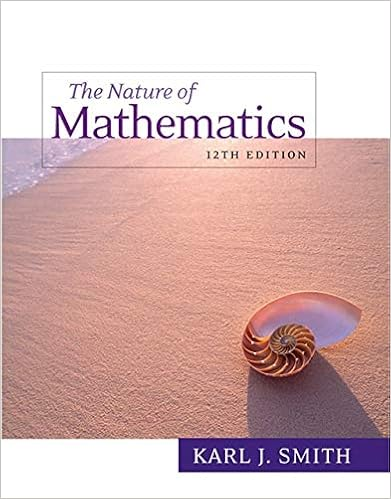# ALG 1-4 Properties of Real Numbers - ALGEBRA 1-2 CHAPTER 1...

• Notes
• 6
• 100% (1) 1 out of 1 people found this document helpful

This preview shows page 1 - 6 out of 6 pages.

##### We have textbook solutions for you!
The document you are viewing contains questions related to this textbook.The document you are viewing contains questions related to this textbook.
Chapter 5 / Exercise 14
Nature of Mathematics
SmithExpert Verified
ALGEBRA 1-2CHAPTER 1SECTION 1-4: PROPERTIES OF REAL NUMBERSOBJECTIVE: To identify and use properties of real numbers 1) Relationships that are always true for real numbers are called properties, which are rules used to rewrite and compare expressions.2) Two algebraic expressions are equivalent expressions if they have same value for all values of the variable(s).. 3) The Commutative Property of Addition and Multiplicationstates the order in which you add or multiply numbers does not change their sum or product.a + b = b + a a b = b a7 + 3 = 3 + 7 12 1/4= 1/412
##### We have textbook solutions for you!
The document you are viewing contains questions related to this textbook.The document you are viewing contains questions related to this textbook.
Chapter 5 / Exercise 14
Nature of Mathematics
SmithExpert Verified
.
36) The product of any number and one is equal to the number, one is called the Multiplicative Identity.
7) The property that states the product of any number
•••